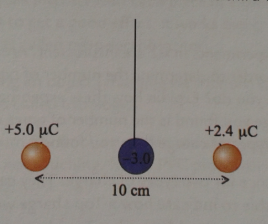# Problem: Two charged balls, one with a charge of +5.0  μC and the other with a charge of +2.4 μC, are placed a distance d = 10.0 cm apart from each other and rigidly held in place. A third ball with a charge of -3.0 μC is dangled from a string between the two charged balls such that it remains in equilibrium. Calculate the distance the third charged ball is from to the +2.4 μC charge.

###### FREE Expert Solution

Coulomb's law:

$\overline{)\begin{array}{rcl}{\mathbit{F}}& {\mathbf{=}}& \frac{{\mathbf{kq}}_{\mathbf{1}}{\mathbf{q}}_{\mathbf{2}}}{{\mathbf{r}}^{\mathbf{2}}}\end{array}}$

$\overline{){\mathbf{x}}{\mathbf{=}}\frac{\mathbf{-}\mathbf{b}\mathbf{±}\sqrt{{\mathbf{b}}^{\mathbf{2}}\mathbf{-}\mathbf{4}\mathbf{a}\mathbf{c}}}{\mathbf{2}\mathbf{a}}}$

$\overline{){{\mathbf{\left(}}{\mathbf{a}}{\mathbf{-}}{\mathbf{b}}{\mathbf{\right)}}}^{{\mathbf{2}}}{\mathbf{=}}{{\mathbf{a}}}^{2}{\mathbf{-}}{\mathbf{2}}{\mathbf{a}}{\mathbf{b}}{\mathbf{+}}{{\mathbf{b}}}^{{\mathbf{2}}}}$

q1 = 5.4 μC(10-6C/1μC) = 5.4 × 10-6 C

q2 = 2.4 μC(10-6C/1μC) = 2.4 × 10-6 C

q3 = -3.0 μC(10-6C/1μC) = -3.0 × 10-6 C

d = 10.0cm(1m/100cm) = 0.1 m

let q2 and q3 be separated by x m

Then, q1 and q3 are separated by d - x = (0.1 - x)

81% (462 ratings)###### Problem Details

Two charged balls, one with a charge of +5.0  μC and the other with a charge of +2.4 μC, are placed a distance d = 10.0 cm apart from each other and rigidly held in place. A third ball with a charge of -3.0 μC is dangled from a string between the two charged balls such that it remains in equilibrium. Calculate the distance the third charged ball is from to the +2.4 μC charge.